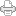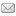## "Floundering Around in the Dark and Actually Stuck"

Posted on Wednesday, November 16, 2011 at 09:09AM

I just finished watching an interesting talk by Ed Witten entitled “Knots and Quantum Theory,” given at the prestigious Institute for Advanced Studies (IAS) at Princeton. Witten more or less describes his exploration of the connection that knot theory has to quantum theory, which serves to exemplify how researchers spend most of their time “floundering around in the dark,” and finding themselves “actually stuck” in their efforts to make sense out of the physical structure of the universe.

I have found the same thing in my little microcosm of theoretical physics research. Only in my case, instead of struggling to understand the monumental and vast complexity of the arcane subjects with which the intellectual giants at IAS work, I struggle to understand the straightforward first four numbers, one, two, three and four. Nevertheless, I too must admit that I spend most of my time floundering in the dark and actually stuck.

For example, for a long time I have tried to connect in my pea brain the LRC’s preon model of the so-called elementary particles of particle physics with the two balls of Larson’s cube (LC); that is, the inner and outer balls defined by the LC, the one contained by it and the one containing it.

These two balls are nested in an intriguing way that can be extended in both “directions” analogous to the way numbers can be extended in the positive and negative “directions.” I had noticed that the radius of the next smaller inner ball (in the “negative direction”), nested inside the radius of the initial inner ball, and is the inverse of the first outer ball, with the inner ball in between them representing unity (to distinguish these three balls, I will refer to them as inverse, unit and outer, from this point on) And since, presumably, this is the relationship of the unit space and unit time oscillations (SUDRs & TUDRs) used to construct the preons of our preon model, it seemed to be a natural conclusion that they could be used to quantify the SUDR and TUDR. However, so far, the attempt to proceed on this basis has only led to floundering and I have been stuck ever since.

At length, I’ve had to conclude that, in spite of tantalizing clues that this is the direction to go, I must be doing something wrong. I must not be thinking about the LC in the correct way and this has caused me to reflect on the curious observation that I made some time ago, that these balls are nothing real. The only real part of the construction is the LC itself. The balls can only exist as some sort of phantom representation of the discrete numbers of the LC. They appear as soon as the LC appears and disappear as soon as the LC disappears, even though their radii are both smaller and larger than the unit magnitude of the LC, regardless of how small or large that unit might be, ad infinitum.

Yet, what I have also known for some time is that the ratio of the inverse and outer balls is a rational number, which relates to the discrete numbers of the LC in a remarkable way, but I think I tried to use that fact in a way that that led me to equate the SUDR and TUDR with the two, inverse, balls directly, when I should have been using their ratio only.

This means that the reciprocal relationship of the SUDR and TUDR is still to be found in the original 1/2+1/1+2/1 = 4/4 equation, not in its equivalent, substituting the square root of 2 or the square root of 3 for the unit. However, both of those square roots are associated with the discrete equation in the sense that their ratio translates the discrete numbers of the LC to the continuous magnitudes of the two, inverse, balls.

For example, the numerical progression of the LC is 2= 8, 4= 64, 6= 216, 8= 512, where the base number is 2 because each dimension has two “directions,” and the exponent is 3 because there are no new phenomena beyond 3 dimensions (Bott’s periodicity  theorem). But just as the LC’s volume expansion is a discrete number, the expansion of its two associated, inverse, balls is a continuous magnitude, given by the volume formula for a ball, V = 4/3 * π * r3

Consequently, since the r of the 2D slice of the unit ball is always 1/2 of the cube root of the LC discrete number, the associated discrete progression of its radius is, 1/2*81/3 = 1, 1/2*641/3 = 2, 1/2*2161/3 = 3, 1/2*5121/3 = 4, and since the r of the 2D slice of the outer ball is always the square root of 2 times the corresponding r of the 2D slice of the unit ball, the discrete progression of its radius is 21/2 * 1 = 21/2, 21/2 * 2 = 81/2, 21/2 * 3 = 181/2, 21/2 * 4 = 321/2.

Now, the question is, what are the corresponding radii of the 2D slice of the inverse balls? The procedure that I have been trying to use depends on the assumption that this radius can be determined by geometric construction: Simply construct a new LC inside the unit ball and take the radius that fits inside it as the next lower radius in the progression. This procedure comes from the fact that each upper radius can be constructed similarly. So, how can it matter what size we choose as the unit size to relate the two inverses to?

However, as I said, this has led to floundering, even though it seems logical. I should note that it was compelling to me for at least two reasons. First, it’s easy to see that the radius of the first ball constructed in this manner is the inverse of the square root of 2, the radius of the outer ball. Second, it allows us to extend the radii in two “directions” from the unit ball, situated between these two, inverse, radii, just as the number 1/1 is situated between the two inverse numbers, 1/2 and 2/1.

In spite of these two sirens, however, I think it’s necessary to resist the temptation to go that way and instead to look at unity as the real part that must be increased. This means that we take the unit progression as 1/1, 2/2, 3/3, …n/n. While this might seem to be a trivial assumption given the fundamental postulates of the RST, the 1/2+1/1+2/1 = 4/4 equation was misleading, since it seemed to imply that the traditional number line, 1/1, 2/1, 3/1, …n/1, should be taken as representing the positive displacement values we need: To the left of 1/1, we have the increasing values of what Larson called time displacements, and to the right we have the increasing values of what he called space displacements, which are the inverse of the time displacements.

This is logical and straightforward, but perhaps it is wrong in the sense that it only succeeds in describing the inverse displacements from 2/2. After all, we can’t get any displacement from 1/1. If this is so, then the next two displacements we have to go to are at the 4/4 and the 6/6 units in the progression, and so on:

1) 2/2 = (1/2)/(1/2)
2) 4/4 = (1/4)/(1/4)
2) 6/6 = (1/6)/(1/6)
.
.
.
n) n/n = (1/n)/(1/n)

The advantage of this line of thinking over the previous is that it brings the mathematical development into conformity with the postulates, and it makes the relationship of the SUDR and TUDR to be inverse in the same sense that space and time are inverse: The quantity on the left is the inverse of the quantity on the right, by virtue of the division symbol; that is, they are not inverse numerically (i.e. both are positive), but they are inversely related in the equation, just as two opposed, but equal, 1D line segments are both positive magnitudes.

It’s also obvious that the 1 in the numerator is not the quantity 1 numerically, but it is the same number that is in the denominator, raised to the power of 3, representing 1 volume unit as a whole, expanded and contracted, as the number 1 represents one cycle of 2π radians in the LST equations. Thus, in terms of space and time dimensions, the discrete progression turned oscillation is different in each dimension. For three dimensions, the volume progression (i.e. volume frequency of oscillation, in units per cycle) is:

2*(23)/2*(20) = 8, 2*(43)/4*(20) = 32, 2*(63)/6*(20) = 72, 2*(83)/8*(20) = 128.

For two dimensions, the frequency is:

2*(22)/2*(20) = 4, 2*(42)/4*(20) = 8, 2*(62)/6*(20) = 12, 2*(82)/8*(20) = 16.

For one dimension, the frequency is:

2*(21)/2*(20) = 2, 2*(41)/4*(20) = 2, 2*(61)/6*(20) = 2, 2*(81)/8*(20) = 2,

which is constant!

To say the least, this has very encouraging implications for the preon combos, but we need continuous magnitudes, not discrete units, since nature expands (contracts) spherically, not cubically. This is where the ratio of the outer ball and its inverse comes in: It serves to translate these discrete mathematical numbers into continuous physical magnitudes, or at least that is what we hope for.

More next time.View Printer Friendly VersionEmail Article to Friend